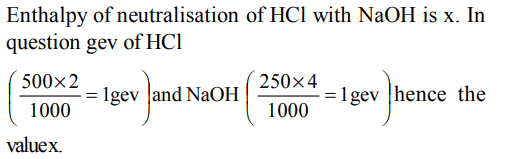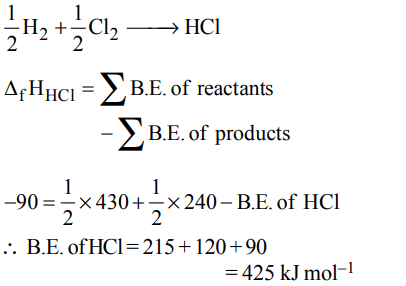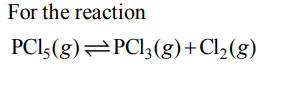## Thermodynamics Questions and Answers Part-7

1. Enthalpy of neutralisation of HCl with NaOH is x. The heat evolved when 500 ml of 2 N HCl are mixed with 250 ml of 4N NaOH will be.
a) 500 x
b) 100x
c) x
d) 10 x

Explanation:2. When 1 M $H_{2}SO_{4}$   is completely neutralised by sodium hydroxide, the heat liberated is 114.64 kJ. What is the enthalpy of neutralisation ?
a) +114.64 kJ
b) –114.64 kJ
c) –57.32 kJ
d) + 57.32 kJ

Explanation:3. The amount of energy released when 20 ml of 0.5 M NaOH are mixed with 100 ml of 0.1 M HCl is x kJ. The heat of neutralisation $\left( kJ mol^{-1}\right)$ is
a) –100x
b) –50x
c) + 100 x
d) +50x

Explanation: In question 0.01 gev of NaOH is being neutralised by 0.01 gev of HCl and value is x kJ, for 1 gev the value is - 100x (exothermic process)

4. The $\triangle H_f^0$   for $CO_{2}\left(g\right), CO \left(g\right) and H_{2}O\left(g\right)$       are –393.5, –110.5 and $–241.8 kJ mol^{-1}$respectively. The standard enthalpy change (in kJ) for the reaction
$CO_{2}\left(g\right)+ H_{2}\left(g\right)\rightarrow CO \left(g\right)+ H_{2}O\left(g\right)$
a) 524.1
b) 41.2
c) –262.5
d) -41.2

Explanation:5. $H_{2}\left(g\right)+ CI_{2}\left(g\right)\rightarrow 2 HCl \left(g\right)\triangle H=- 44 kcal$
$2 Na\left(s\right)+ 2 HCl\left(g\right)\rightarrow 2NaCl \left(s\right)+H_{2}\left(g\right)\triangle H=-152kcal$
For the reaction
$Na\left(s\right)+\frac{1}{2} Cl_{2}\left(g\right)\rightarrow NaCl \left(s\right)\triangle H=?$
a) –180 kcal
b) –196 kcal
c) –98 kcal
d) 54 kcal

Explanation:6. The heat required to decompose a compound into its elements is equal to the heat evolved when the compound is formed from its elements. This is in accordance with :
a) Lavosier and laplace law
b) Second law of thermodynamics
c) Joule- Thomson law
d) Kirchoff’s law

Explanation: Lavoisier and Laplace law

7. Consider the following reactions:
(i) $H^{+}\left(aq\right)+OH^{-}\left(aq\right)\rightarrow H_{2}O\left(l\right)$
$\triangle H=-X_{1}kJ mol^{-1}$
(ii) $H_{2}\left(g\right)+\frac{1}{2}O_{2}\left(g\right)\rightarrow H_{2}O\left(l\right)$
$\triangle H=-X_{2}kJ mol^{-1}$
(iii) $CO_{2}\left(g\right)+H_{2}\left(g\right)\rightarrow CO\left(g\right)+ H_{2}O$
$\triangle H=-X_{3}kJ mol^{-1}$
(iv) $C_{2}H_{2}\left(g\right)+\frac{5}{2}O_{2}\left(g\right)\rightarrow 2CO_{2}\left(g\right)+ H_{2}O\left(l\right)$
$\triangle H=+4X_{4}kJ mol^{-1}$
Enthalpy of formation of $H_{2}O\left(l\right)$   is
a) $+X_{3}kJ mol^{-1}$
b) $-X_{4}kJ mol^{-1}$
c) $+X_{1}kJ mol^{-1}$
d) $-X_{2}kJ mol^{-1}$

Explanation: This reaction shows the formation of H2O, and the – X2 represents the enthalpy of formation of H2O because as the definition suggests that the enthalpy of formation is the heat evolved or absorbed when one mole of substance is formed from its constituent elements.

8. Given that bond energies of H – H and Cl – Cl are 430 kJ $mol^{-1}$  and 240 kJ $mol^{-1}$   respectively and $\triangle_{f}H$   for HCl is $-90 kJ mol^{-1}$  , bond enthalpy of HCl is
a) $380 kJ mol^{-1}$
b) $425 kJ mol^{-1}$
c) $245 kJ mol^{-1}$
d) $290 kJ mol^{-1}$

Explanation:9. Which of the following are not state functions ?
(I) q + w
(II) q
(III) w
(IV) H - TS
a) (I) and (IV)
b) (II), (III) and (IV)
c) (I), (II) and (III)
d) (II) and (III)

Explanation: We know that heat (q) and work (w) are not state functions but (q + w) is a state function. H – TS (i.e. G) is also a state function. Thus II and III are not state functions so the correct answer is option (d).

10. For the gas phase reaction
$PCl_{5}\left(g\right)\rightleftharpoons PCl_{3}\left(g\right)+Cl_{2}\left(g\right)$
which of the following conditions are correct ?
a) $\triangle H=0$   and $\triangle S<0$
b) $\triangle H>0$   and $\triangle S>0$
c) $\triangle H<0$   and $\triangle S<0$
d) $\triangle H>0$   and $\triangle S<0$The reaction given is an example of decomposition reaction and we know that decomposition reactions are endothermic in nature, i.e., $\triangle$H > 0.
$\triangle$n = (1+1) – 1= +1
Hence more number of gaseous molecules are present in products which shows more randomness i.e., $\triangle$S > 0 ($\triangle$S is positive)